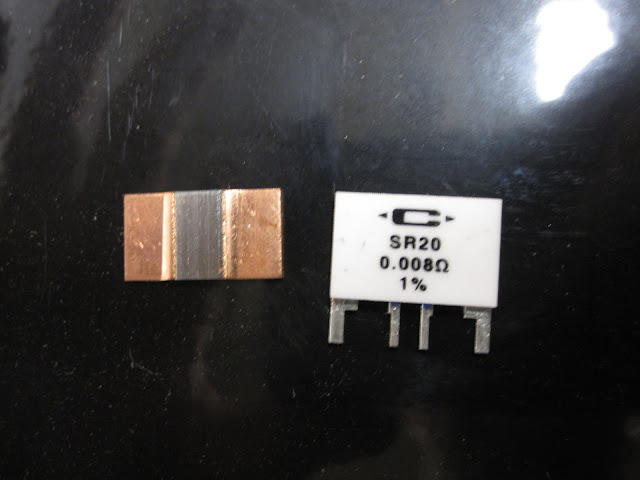## DIY Milliohm Meter

25 08 2011

I asked Joe Khachan this question:

Did you go to great lengths to reduce the resistance of the coil path?

He responded:

Yes we try and use a thick enough wire so that the resistance is small but it cannot be too thick in order to be able to have many turns. More importantly is the contact resistance when connecting to the wires. We try to keep this low. From this perspective, a milliOhm meter is helpful.

Milliohm meters start around \$200 on ebay. Too much.

Then I found this excellent tutorial on Kelvin (4-wire) resistance measurement.

Luckily I had a current sensing resistor on hand for the current reading:I used the resistor on the right.

So current sense resistor plus two voltmeters and BINGO: milliohm meter. Results:

The coil path is 0.35Ω.

The dummy coil is 0.185Ω

As a calibration I tested the 0.001Ω resistor. Result: 0.00088888

Now I have excellent tool to troubleshoot resistance in the coil path. AWESOME.

## How to Read an Oscilloscope

25 08 2011

Here is a quick tutorial on how to read an oscilloscope.

Voltage increases as you go up the screen.

Time passes from left to right.

The three numbers circled below are the keys.In this example:

1V  means the distance between each gridline bottom to top  represents 1 volt.

500µs means the distance between each gridline left to right represents 500 microseconds.

2.160V is the voltage between two lines I manually adjust. This is called a cursor.

The small cross circled on the lower left indicates zero volts.

Those are the basics of reading a ‘scope.